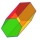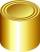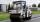# Area of the shape - 9th grade (14y) - math problems

#### Number of problems found: 470

• The cylinder 2Find the volume and the lateral area of a cylinder of height 12 inches and a base radius of 4 inches.
• HexagonCalculate the regular hexagonal prism's surface whose base edge a = 12cm and side edge b = 3 dm.
• SeatHow much m² of fabric do we need to sew a 50cm-shaped cube-shaped seat if 10% of the material we add to the folds?
• Content area and percentsDetermine what percentage is smaller cube surface, when the surface area of the wall decreases by 25%.
• Reservoir6 m long reservoir has a diameter of 2.2 m. What is its surface area in square meters?
• The resistanceWhat is the resistance of an aluminum wire 0.2 km long and 10 mm in diameter?
• RollerRoller has a diameter of 0.96 m and a width 169 cm. How many m2 of road level when he turns 42-times?
• Pyramid cutWe cut the regular square pyramid with a parallel plane to the two parts (see figure). The volume of the smaller pyramid is 20% of the volume of the original one. The bottom of the base of the smaller pyramid has a content of 10 cm2. Find the area of the
• RollerCylinder shell has the same content as one of its bases. Cylinder height is 15 dm. What is the radius of the base of the cylinder?
• Circular poolThe base of the pool is a circle with a radius r = 10 m, excluding a circular segment that determines the chord length 10 meters. The pool depth is h = 2m. How many hectoliters of water can fit into the pool?

We apologize, but in this category are not a lot of examples.
Do you have an exciting math question or word problem that you can't solve? Ask a question or post a math problem, and we can try to solve it.

We will send a solution to your e-mail address. Solved examples are also published here. Please enter the e-mail correctly and check whether you don't have a full mailbox.

Examples of area of plane shapes. Examples for 9th grade.